# B007 – Gas VCF

## Description

This block calculates the uncertainty in the Volume Correction Factor (VCF) for gas.

## Options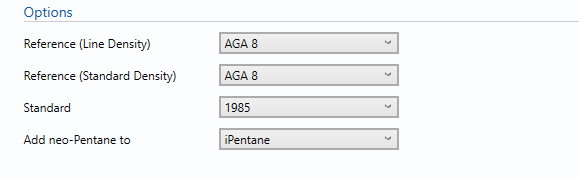### Reference (Line Density)

AGA 8 is the reference used by KELTON UNCERTAINTYPLUS to compute the gas line density.

### Reference (Standard Density)

• AGA 8
• ASTM D3588
• ISO 6976

This option determines which Reference is used for the calculation of Standard Density.

### Standard

For AGA 8:

• 1985
• 1994

For ISO 6976:

• 1983
• 1989
• 1995

Select the year to which the reference is been used for the calculation.

• i-Pentane
• n-Pentane

This option is used to determine which component any neo-pentane will be added to.

Note: This option is only available in AGA 8

### GPA 2145 physical constants reference table

• 1989
• 2000
• 2003
• 2009

Select the table to be used to get the physical constant.

Note: This option is only available in ASTM D3588.

### Reference Temperature (Combusting Metering)

• 25/0
• 15/0
• 0/0
• 15/15

Reference Temperature for combusting and metering.

Note: This option is only available in ISO 6976.

## Inputs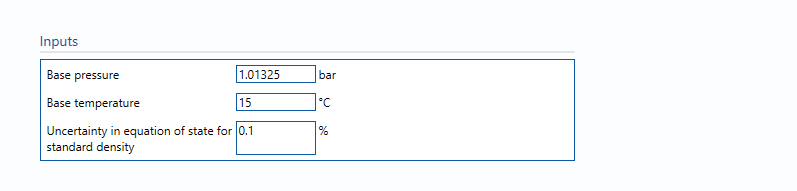#### Base pressure

The pressure at base conditions.

#### Base temperature

The temperature at base conditions.

#### Uncertainty in equation of state for standard density

Uncertainty in the equation of state. Guidelines on determining this value can be found in the relevant standard.

Note: that the uncertainties given in AGA8:1994, Figure 1 are only valid for the “normal” range of compositions given in the standard; for compositions in the “extended” range the uncertainty will be higher, but the standard does not specify how much higher. Engineering judgement should be used here, however it is recognised that this is difficult at best, due to lack of data.

## Uncertainty Budget

The uncertainty calculation is detailed in the uncertainty budget table giving a break down of how the overall uncertainty is calculated. The values input into the uncertainty budget are taken from the module inputs and calculations from other blocks along with the uncertainty in the equation of state as input in this block.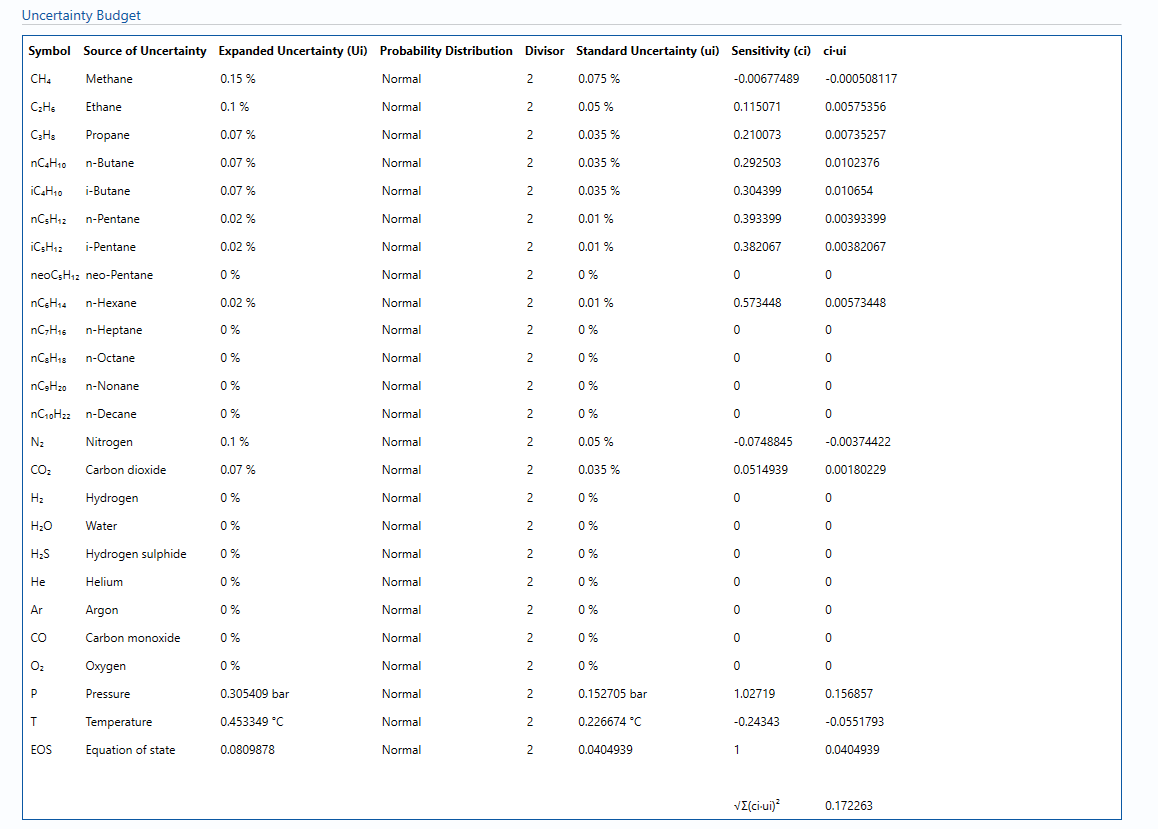Uncertainties associated with these values are taken in as the Expanded Uncertainties. The Standard Uncertainty is calculated from dividing the Expanded Uncertainty by the coverage factor which is determined by the probability distribution that best suits that uncertainty component.

The standard uncertainty is then multiplied by the sensitivity value then squared. This is done for each parameter that contributes to the overall uncertainty in the Volume Correction Factor. The Standard Uncertainty in Volume Correction Factor is the square root of the sum of each component variance as shown in the following equation: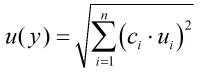### Calculated Uncertainty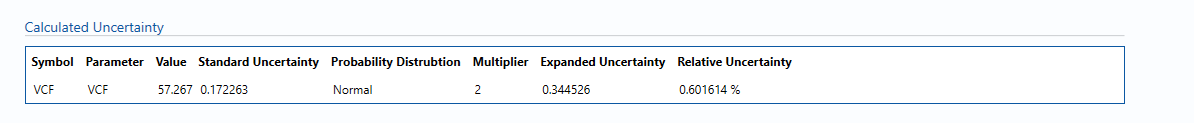The Expanded Uncertainty is the Standard Uncertainty multiplied by the coverage factor (k). The coverage factor is defaulted to k = 2 (equivalent to a confidence level of approximately 95%).

The Relative Uncertainty is the Expanded Uncertainty divided by calculated VCF.

### Standards

ISO 5168:2005 – Measurement of fluid flow – Procedures for the evaluation of uncertainties

ISO Guide 98-3 – Uncertainty of measurement – Part 3: Guide to the expression of uncertainty in measurement (GUM:1995) (2008)

AGA Report No. 8 – Compressibility and Supercompressibility of Natural Gas and Other Related Hydrocarbon Gases (December 1985)

AGA Report No. 8 – Compressibility Factors of Natural Gas and Other Related Hydrocarbon Gases (2nd Printing July 1994)

ISO 12212-2:2006 – Natural Gas – Calculation of compression factor – Part 2: Calculation using molar composition analysis

### KCCL Calculations

C156 – AGA8 1985/94 – Gas Density and Compressibility

### Uncertainty Blocks

B004 – Composition

Back to Uncertainty Modules